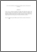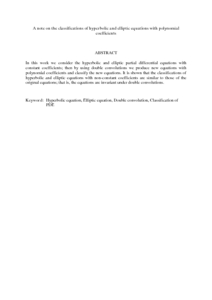# A note on the classifications of hyperbolic and elliptic equations with polynomial coefficients

## Citation

Kilicman, Adem and Eltayeb, Hassan (2008) A note on the classifications of hyperbolic and elliptic equations with polynomial coefficients. Applied Mathematics Letters, 21 (11). pp. 1124-1128. ISSN 0893-9659

## Abstract

In this work we consider the hyperbolic and elliptic partial differential equations with constant coefficients; then by using double convolutions we produce new equations with polynomial coefficients and classify the new equations. It is shown that the classifications of hyperbolic and elliptic equations with non-constant coefficients are similar to those of the original equations; that is, the equations are invariant under double convolutions.Preview
PDF (Abstract)
A note on the classifications of hyperbolic and elliptic equations with polynomial coefficients.pdfView Item